# Use ggplot to draw a single curve

As an example, consider the function

\[f(r) = 1/r\]

We may want to plot values of \(r\) in the interval \((1,5)\). Lets make a data frame with one column:

``````DF1 <- data.frame( r = 1:5 )
DF1``````
``````##   r
## 1 1
## 2 2
## 3 3
## 4 4
## 5 5``````

Now compute the result and put it into a new column in the data frame:

``````DF1\$t <- 1 / DF1\$r
DF1``````
``````##   r         t
## 1 1 1.0000000
## 2 2 0.5000000
## 3 3 0.3333333
## 4 4 0.2500000
## 5 5 0.2000000``````

Now we plot data in `DF1`, mapping `r` to the x-axis, `t` to the y-axis, and drawing lines between the x,y pairs:

``````library(ggplot2)
ggplot( DF1, aes( x = r, y = t ) ) +
geom_line()``````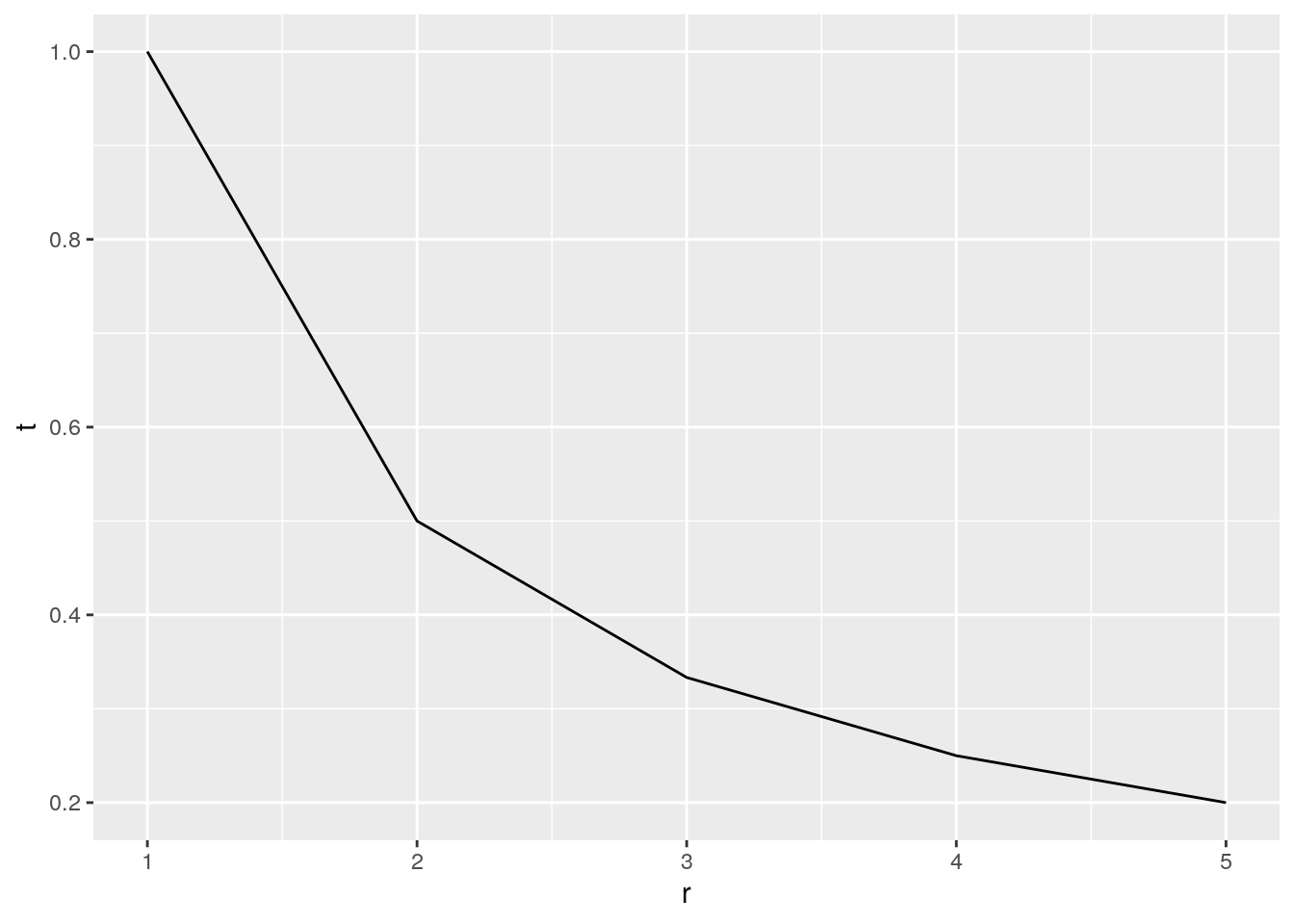Figure 1-1. Simple line plot

We can change this to plot points instead:

``````ggplot( DF1, aes( x = r, y = t ) ) +
geom_point()``````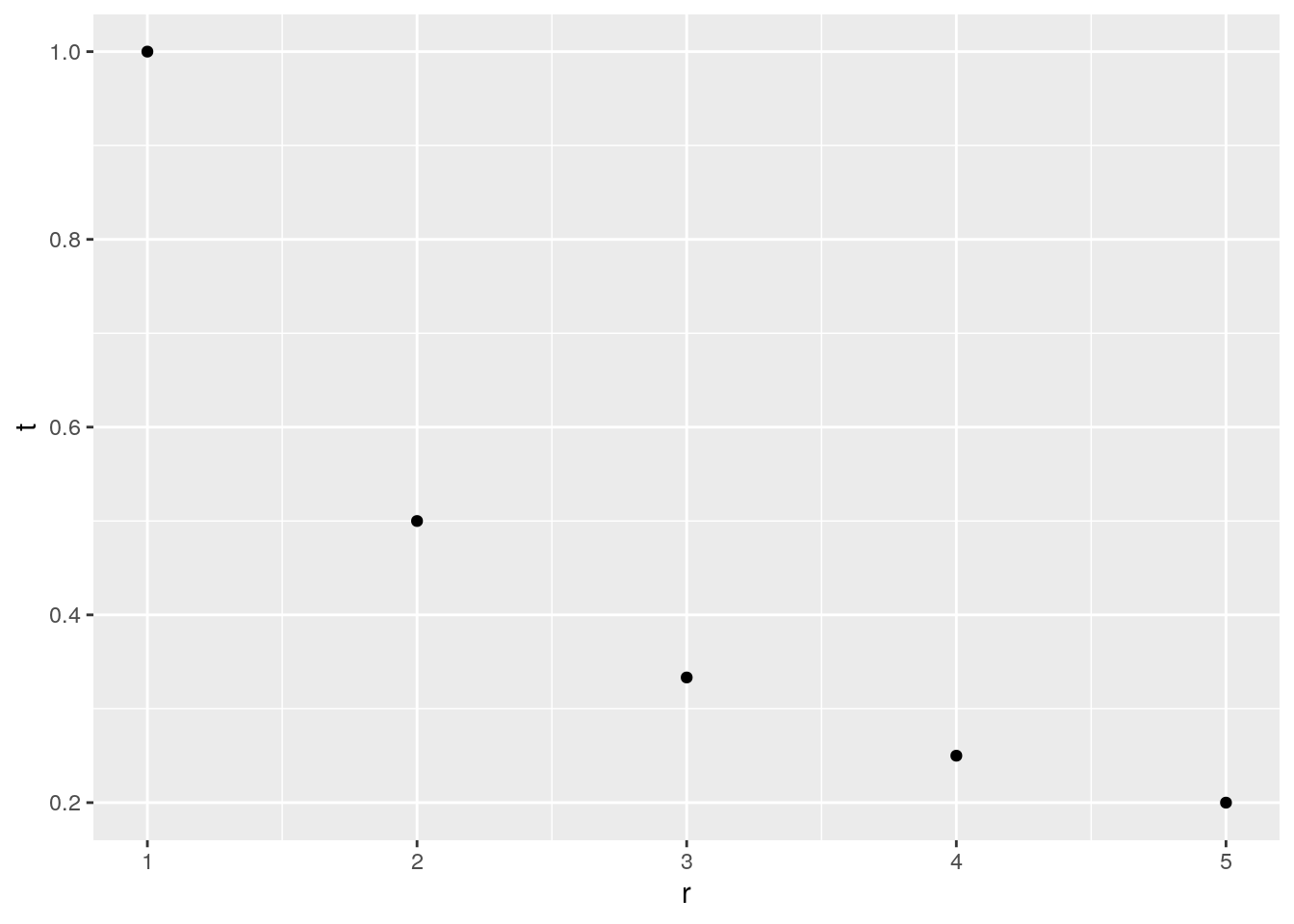Figure 1-2. Simple points plot

or we can overlay them and change the size and color of the points:

``````ggplot( DF1, aes( x = r, y = t ) ) +
geom_line() +
geom_point( size = 3, color = "red" )``````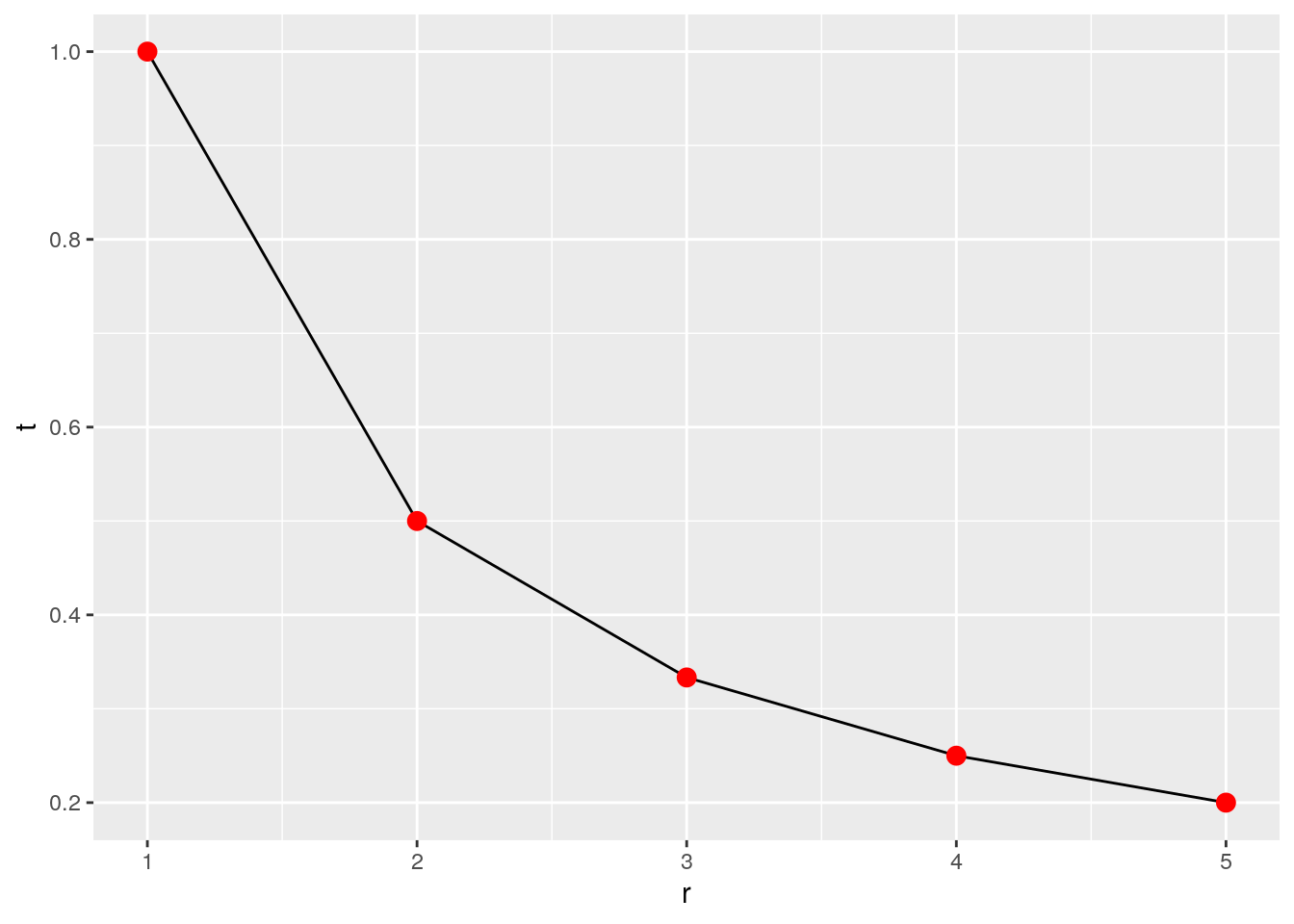Figure 1-3. Simple line plot with points having fixed size and color

but we cannot get a legend for the linesize or color when we specify them on the fly. Rather, we have to map columns of category data to the color and size:

``````DF1\$Test <- "First Test"
DF1\$Data <- "Samples"
DF1``````
``````##   r         t       Test    Data
## 1 1 1.0000000 First Test Samples
## 2 2 0.5000000 First Test Samples
## 3 3 0.3333333 First Test Samples
## 4 4 0.2500000 First Test Samples
## 5 5 0.2000000 First Test Samples``````
``````ggplot( DF1, aes( x = r, y = t ) ) +
geom_line() +
geom_point( mapping = aes( size = Test, color = Data ) )``````
``## Warning: Using size for a discrete variable is not advised.``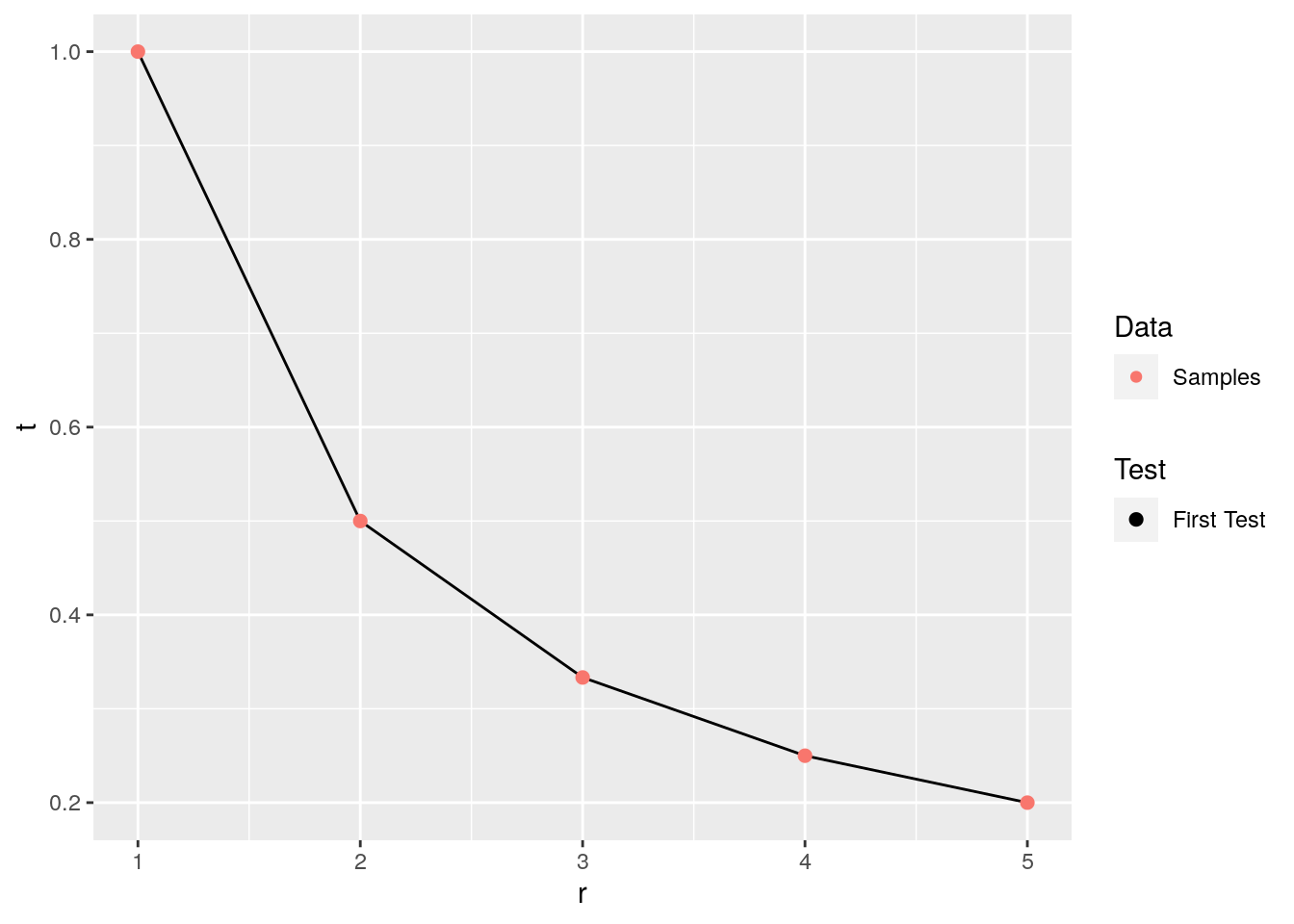Figure 1-4. Simple line plot with points and auto legend

Finally, if we want to control how column data are translated into size and colour, we have to make sure discrete variables are setup as factors first, and then adjust their “scales”:

``````DF1\$Testf <- factor( DF1\$Test, levels = "First Test" )
DF1\$Dataf <- factor( DF1\$Data, levels = c( "Samples", "Function" ) )
ggplot( DF1, aes( x = r, y = t ) ) +
geom_line() +
geom_point( mapping = aes( size = Testf, color = Dataf ) ) +
scale_size_manual( name = "Test", values = 3 ) +
scale_color_manual( name = "Data", values = "red" )``````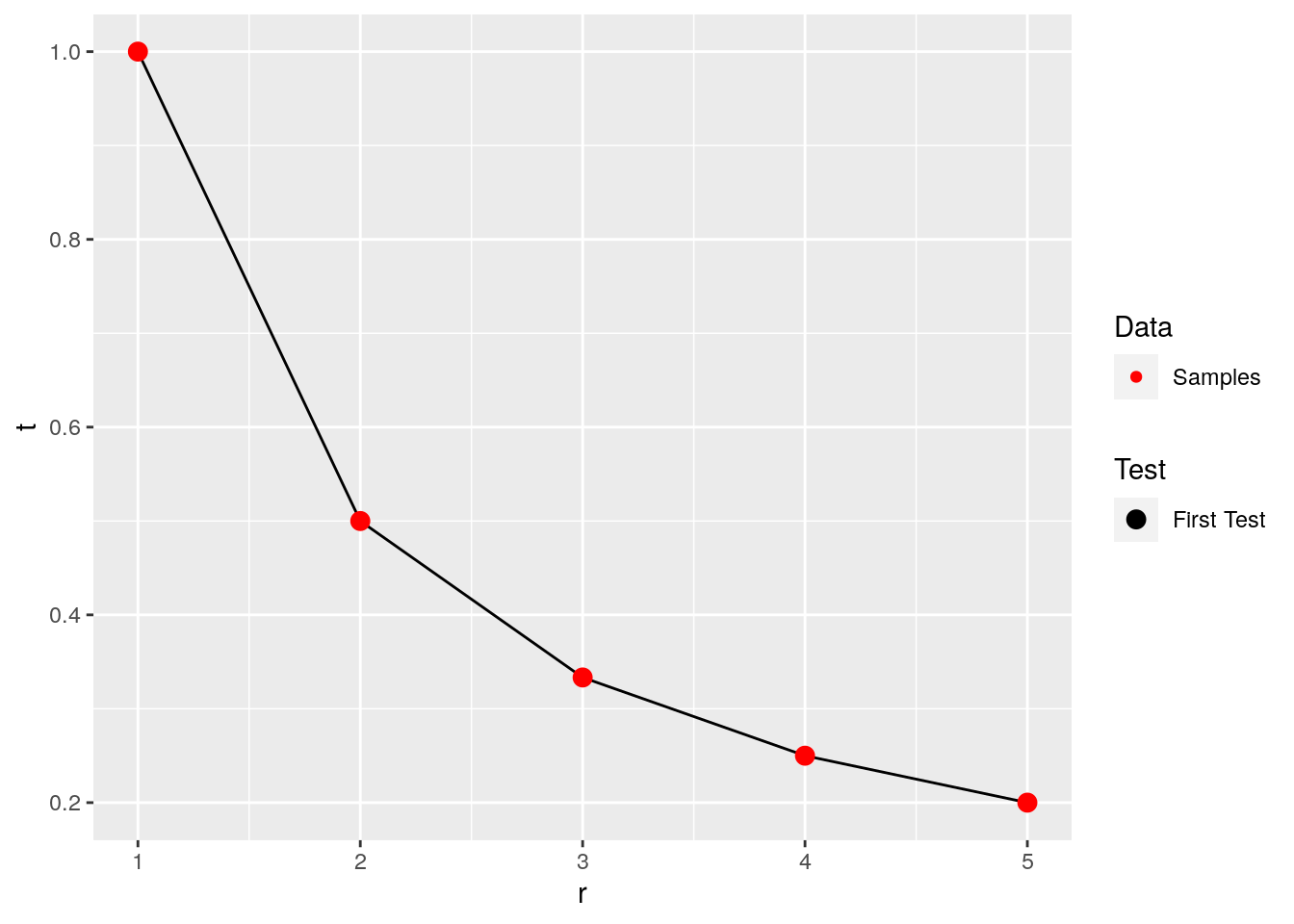Figure 1-5. Simple line plot with points and modified legend

The values arguments indicate by position the graphical value corresponding to each factor level.

You can read about factors in the Introduction to R manual that comes with R. They look a lot like character vectors, but are actually vectors of integers that are automatically used as indexes in a (usually shorter) character vector of “levels”. They tend to be useful in the final stages of analysis or display, but are not well suited for combining data sources… for example, don’t try concatenating factor vectors.

# Plotting functions with ggplot

While normally functions are evaluated and the data are plotted, sometimes you just want a quick way to overlay what a function looks like on your graph of data points. The `stat_function` function handles this:

``````g <- function( x ) {
1 / x
}
ggplot( DF1, aes( x = r, y = t ) ) +
geom_line() +
geom_point( mapping = aes( size = Test, color = Data ) ) +
stat_function( fun = g, mapping = aes( color = "Function" ) ) +
scale_size_manual( values = 3 ) +
scale_color_manual( values = c( "blue", "red" ) ) +
xlab( "r (km/h)" ) +
ylab( "t (h)" )``````
``## Warning: `mapping` is not used by stat_function()``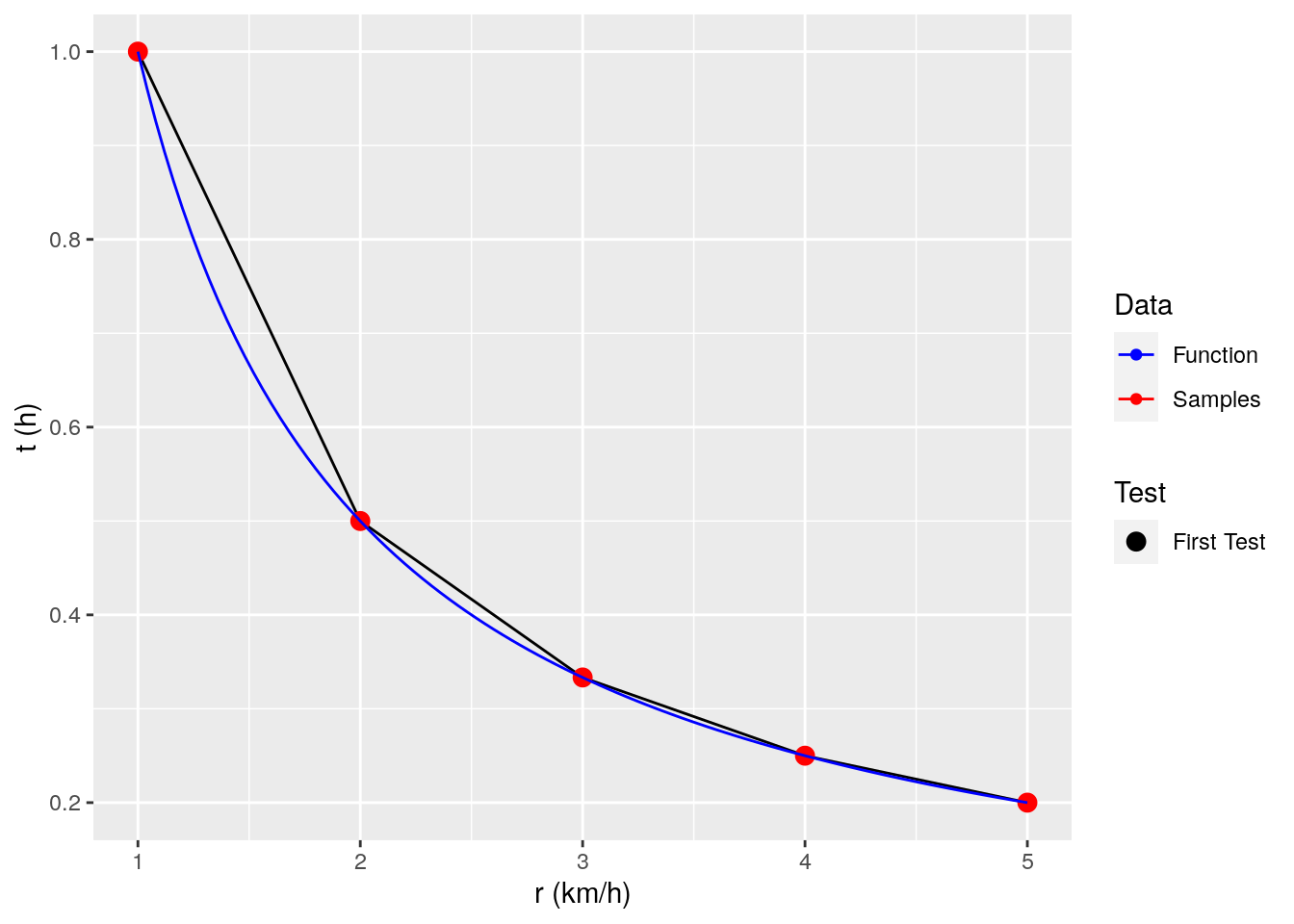Figure 2-1. Simple line plot with points and modified legend and function

(Optional detail: notice that factors are not being specified here for size and color, because the legend applies to all data shown on the plot, and the `stat_function` option is automatically generating and adding a bunch of points to the plot, and their size and color must be assigned on the fly. To do this, `ggplot` appends new records to the mapped data with color column values for those records set to “Function”. Since concatenating factors does not work in general, the character vectors are concatenated and the unique strings are identified and sorted alphabetically to make the “effective” levels for the color factor. Since it is done internally, there is no way for us to specify the order of those levels outside ggplot. This is why the `stat_function` function is not used very often… better control over the results can be obtained by generating all of the points to plot before giving them to ggplot.)

# Use ggplot to draw multiple curves

Now consider the function

\[f(r) = d/r\]

where \(d=1,2,3\) is a distance, \(r\) is a rate, and \(t=f(r)\) is time. We may want to plot values of \(r\) in the interval \((1,5)\) for each value of \(d\).

The direct way to make a data frame that contains combinations of x,y points is:

``````x <- 1:5
DF2 <- data.frame( d = rep( c( 1, 2, 3 )
, each = length( x ) )
, r = c( x, x, x )
)
str( DF2 )``````
``````## 'data.frame':    15 obs. of  2 variables:
##  \$ d: num  1 1 1 1 1 2 2 2 2 2 ...
##  \$ r: int  1 2 3 4 5 1 2 3 4 5 ...``````
``head( DF2 )``
``````##   d r
## 1 1 1
## 2 1 2
## 3 1 3
## 4 1 4
## 5 1 5
## 6 2 1``````

Now we have a data frame with all combinations of the inputs needed for the function. (Note that there are more compact ways to build such combinations of inputs, such as the `expand.grid` function that will be discussed later.) We can augment this data frame with a new column that contains the computed results:

``````f <- function( r, d ) {
d/r
}
DF2\$t <- f( DF2\$r, DF2\$d )
``````##   d r         t
## 1 1 1 1.0000000
## 2 1 2 0.5000000
## 3 1 3 0.3333333
## 4 1 4 0.2500000
## 5 1 5 0.2000000
## 6 2 1 2.0000000``````

Now we have a data frame with both input values and the corresponding output values. Now lets make a first try at the graph:

``````ggplot( DF2, aes( x = r, y = t, color = d ) ) +
geom_line()``````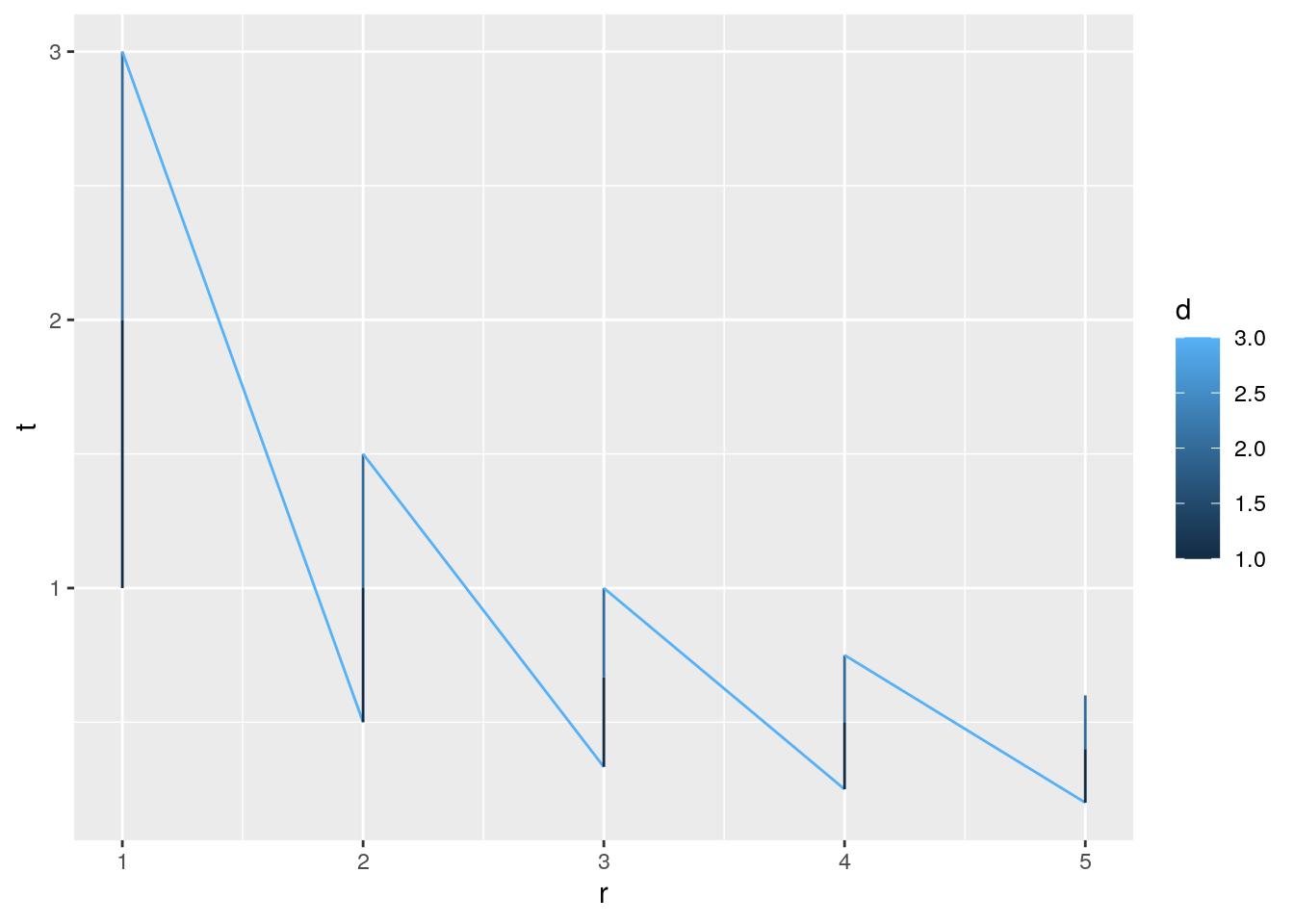Figure 3-1. Multi-curve continuous color

Not quite what we would have hoped. The problem is that we have mapped color to a continuous variable (numeric). We can fix this by changing `d` to a discrete “factor” variable:

``````DF2\$df <- factor( DF2\$d )
str( DF2 )``````
``````## 'data.frame':    15 obs. of  4 variables:
##  \$ d : num  1 1 1 1 1 2 2 2 2 2 ...
##  \$ r : int  1 2 3 4 5 1 2 3 4 5 ...
##  \$ t : num  1 0.5 0.333 0.25 0.2 ...
##  \$ df: Factor w/ 3 levels "1","2","3": 1 1 1 1 1 2 2 2 2 2 ...``````

Now plot a second try using the factor:

``````ggplot( DF2, aes( x = r, y = t, color = df ) ) +
geom_line()``````